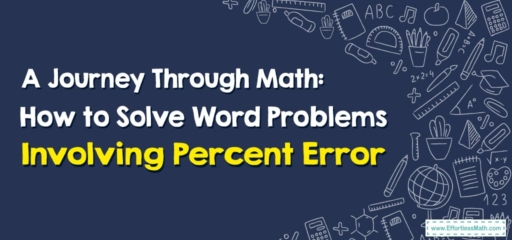# A Journey Through Math: How to Solve Word Problems Involving Percent Error

Hello, mathematical explorers!

As we embark on our adventure today, our map takes us through the challenging terrain of word problems involving percent error. Pack your compass and buckle up, it's time to navigate through these mathematical landscapes!## 1. Starting Point: Understanding Percent Error

Before we hit the trail, let’s familiarize ourselves with the concept:

• Percent Error: This is a measure of how inaccurate a value is, compared to the actual or true value. It’s calculated as the absolute difference between the estimated value and the true value, divided by the true value, all multiplied by $$100$$$$\%$$.

## 2. The Expedition: Solving Word Problems Involving Percent Error

Now that we’ve oriented ourselves, let’s proceed with our exploration!

### Travel Guide: Solving Word Problems Involving Percent Error

#### Step 1: Identify the Estimated and True Values

First, locate the estimated and true values in the problem.

#### Step 2: Calculate the Difference

Next, find the difference between these values by subtracting the estimated value from the true value. Remember to take the absolute value, as we’re interested in the size of the error, not its direction.

#### Step 3: Divide by the True Value

Then, divide this difference by the true value to find the proportion of the error.

#### Step 4: Multiply by 100%

Lastly, multiply the result by $$100$$$$\%$$ to express the error as a percentage.

Consider this problem: A travel guide estimated that a journey would take $$2$$ hours, but it actually took $$2.5$$ hours. What is the percent error?

1. Identify the Estimated and True Values: The estimated time is $$2$$ hours and the true time is $$2.5$$ hours.
2. Calculate the Difference: The difference is $$|2.5 \ – 2| = 0.5$$ hours.
3. Divide by the True Value: The proportion of the error is $$\frac{0.5}{2.5}= 0.2$$.
4. Multiply by $$100$$$$\%$$: The percent error is $$0.2\times100$$$$\%$$$$= 20$$$$\%$$.

Congratulations, mathematical explorers! You’ve successfully navigated through the realm of percent error. Remember, every journey makes us stronger and more skilled in navigating mathematical problems. Until our next adventure, safe travels!

### What people say about "A Journey Through Math: How to Solve Word Problems Involving Percent Error - Effortless Math: We Help Students Learn to LOVE Mathematics"?

No one replied yet.

X
30% OFF

Limited time only!

Save Over 30%

SAVE $5 It was$16.99 now it is \$11.99